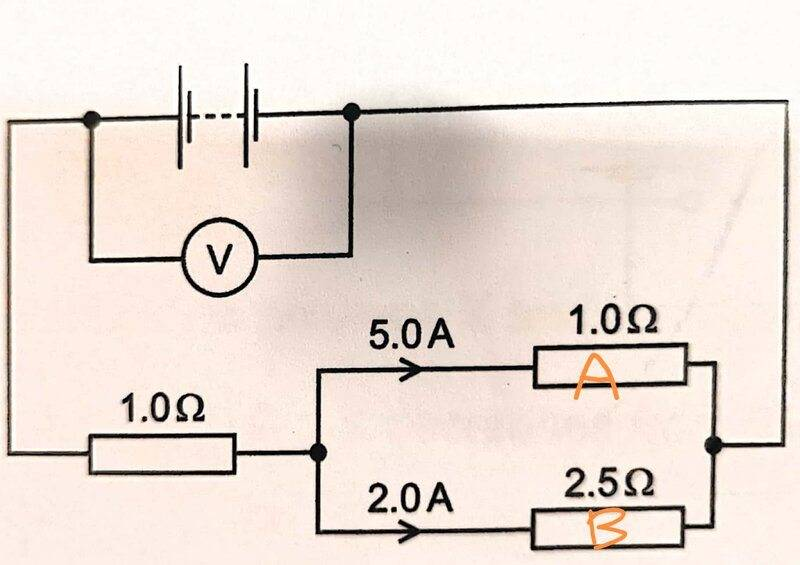# Potential difference calculation

ellieee
Homework Statement:
for the 2 resistors in parallel, is is true that only need to find p.d across one of them (eg. (5.0)(1.0)=5.0V) to determine the p.d across both parallel resistors ? in this case (2.0.)(2.5) = (5.0)(1.0), but what If the p.d across resistor A not equal to that of B, then which resistor do you calculate the p.d of?
Relevant Equations:
V=IR#### Attachments

Staff Emeritus
Homework Helper
Gold Member
That cannot happen.

•ellieee
•